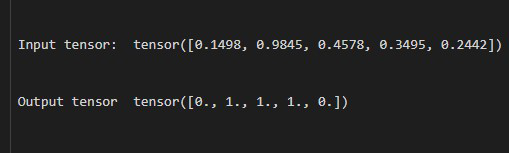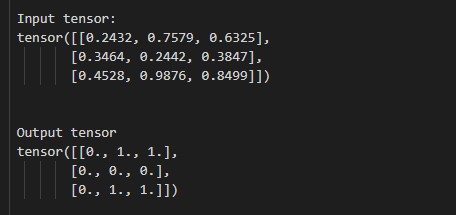GFG App
Open AppBrowser
Continue

# How to Draw Binary Random Numbers (0 or 1) from a Bernoulli Distribution in PyTorch?

In this article, we discuss how to draw Binary Random Numbers (0 or 1) from a Bernoulli Distribution in PyTorch.

## torch.bernoulli() method

torch.bernoulli() method is used to draw binary random numbers (0 or 1) from a Bernoulli distribution. This method accepts a tensor as a parameter, and this input tensor is the probability of drawing 1. The values of the input tensor should be in the range of 0 to 1. This method returns a tensor that only has values 0 or 1 and the size of this tensor is the same as the input tensor. Let’s have a look at the syntax of the given method:

Syntax: torch.bernoulli(input)

Parameters:

• input (Tensor): the input tensor containing the probabilities of drawing 1.

Returns: it will returns a tensor that only has values 0 or 1 and the size of this tensor is the same as the input tensor.

### Example 1

In this example, we draw Binary Random Numbers (0 or 1) from a Bernoulli Distribution using a 1-D tensor.

## Python3

 `# Import required library` `import` `torch`   `# create a tensor containing the ` `# probability of drawing 1.` `tens ``=` `torch.tensor([``0.1498``, ``0.9845``, ``0.4578``, ` `                     ``0.3495``, ``0.2442``])` `print``(``" Input tensor: "``, tens)`   `# Draw random numbers (0,1)` `random_num ``=` `torch.bernoulli(tens)`   `# display result` `print``(``" Output tensor "``, random_num)`

Output:### Example 2

In this example, we estimate the gradient of a function for a 2-D tensor.

## Python3

 `# Import required library` `import` `torch`   `# create a tensor containing the` `# probability of drawing 1.` `tens ``=` `torch.tensor([[``0.2432``, ``0.7579``, ``0.6325``],` `                     ``[``0.3464``, ``0.2442``, ``0.3847``],` `                     ``[``0.4528``, ``0.9876``, ``0.8499``], ])` `print``(``"\n Input tensor: \n"``, tens)`   `# Draw random numbers (0,1)` `random_num ``=` `torch.bernoulli(tens)`   `# display result` `print``(``"\n Output tensor \n"``, random_num)`

Output:My Personal Notes arrow_drop_up